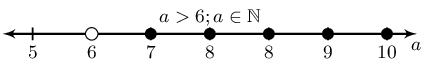Home Practice
For learners and parents For teachers and schools
Textbooks
Full catalogue
Pricing SupportLog in

We think you are located in United States. Is this correct?

# 4.7 Solving linear inequalities

## 4.7 Solving linear inequalities (EMA3H)

A linear inequality is similar to a linear equation in that the largest exponent of a variable is $$\text{1}$$. The following are examples of linear inequalities.

\begin{align*} 2x + 2 & \le 1 \\ \frac{2 - x}{3x + 1} & \ge 2 \\ \frac{4}{3}x - 6 & < 7x + 2 \end{align*}

The methods used to solve linear inequalities are similar to those used to solve linear equations. The only difference occurs when there is a multiplication or a division that involves a minus sign. For example, we know that $$8>6$$. If both sides of the inequality are divided by $$-\text{2}$$, then we get $$-4>-3$$, which is not true. Therefore, the inequality sign must be switched around, giving $$-4<-3$$.

In order to compare an inequality to a normal equation, we shall solve an equation first.

Solve $$2x + 2 = 1$$:

\begin{align*} 2x + 2 & = 1 \\ 2x & = 1 - 2 \\ 2x & = -1 \\ x & = -\frac{1}{2} \end{align*}

If we represent this answer on a number line, we get:

Now let us solve for $$x$$ in the inequality $$2x + 2 \le 1$$:

\begin{align*} 2x + 2 & \le 1 \\ 2x & \le 1 - 2 \\ 2x & \le -1 \\ x & \le -\frac{1}{2} \end{align*}

If we represent this answer on a number line, we get:

We see that for the equation there is only a single value of $$x$$ for which the equation is true. However, for the inequality, there is a range of values for which the inequality is true. This is the main difference between an equation and an inequality.

Remember: when we divide or multiply both sides of an inequality by a negative number, the direction of the inequality changes. For example, if $$x<1$$, then $$-x>-1$$. Also note that we cannot divide or multiply by a variable.

The following video provides an introduction to linear inequalities.

Video: 2FGH

### Interval notation (EMA3J)

Examples:

 $$\left(4;12\right)$$ Round brackets indicate that the number is not included. This interval includes all real numbers greater than but not equal to $$\text{4}$$ and less than but not equal to $$\text{12}$$. $$\left(-\infty ;-1\right)$$ Round brackets are always used for positive and negative infinity. This interval includes all real numbers less than, but not equal to $$-\text{1}$$. $$\left[1;13\right)$$ A square bracket indicates that the number is included. This interval includes all real numbers greater than or equal to $$\text{1}$$ and less than but not equal to $$\text{13}$$.

It is important to note that this notation can only be used to represent an interval of real numbers.

We represent the above answer in interval notation as $$\left(-\infty ; -\frac{1}{2}\right]$$

## Worked example 17: Solving linear inequalities

Solve for $$r$$:

$6 - r > 2$

Represent the answer on a number line and in interval notation.

### Rearrange and solve for $$r$$

\begin{align*} -r & > 2 - 6 \\ -r & > -4 \end{align*}

### Multiply by $$-\text{1}$$ and reverse inequality sign

$r < 4$

### Represent the answer in interval notation

$\left(-\infty ; 4\right)$

## Worked example 18: Solving linear inequalities

Solve for $$q$$:

$4q + 3 < 2(q + 3)$

Represent the answer on a number line and in interval notation.

### Expand the bracket

\begin{align*} 4q + 3 & < 2(q + 3) \\ 4q + 3 & < 2q + 6 \end{align*}

### Rearrange and solve for $$q$$

\begin{align*} 4q + 3 & < 2q + 6 \\ 4q - 2q & < 6 - 3 \\ 2q & < 3 \end{align*}

### Divide both sides by $$\text{2}$$

\begin{align*} 2q & < 3 \\ q & < \frac{3}{2} \end{align*}

### Represent the answer in interval notation

$$\left(-\infty ; \frac{3}{2}\right)$$
temp text

## Worked example 19: Solving compound linear inequalities

Solve for $$x$$:

$5 \le x + 3 < 8$

Represent the answer on a number line and in interval notation.

### Subtract $$\text{3}$$ from all the parts of the inequality

$\begin{array}{ccccc} 5 - 3 & \le & x + 3 - 3 & < & 8 - 3 \\ 2 & \le & x & < & 5 \end{array}$

### Represent the answer in interval notation

$$\left[2 ; 5\right)$$
temp text
Textbook Exercise 4.6

Look at the number line and write down the inequality it represents.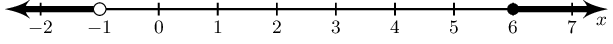$$x < -1 \text{ and } x \ge 6 ; x \in \mathbb{R}$$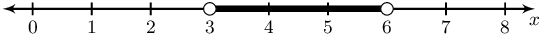$$3 < x < 6 ; x \in \mathbb{R}$$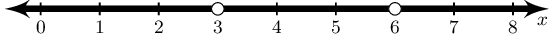$$x \neq 3 ; x \neq 6 ; x \in \mathbb{R}$$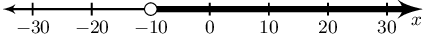$$x > -10 ; x \in \mathbb{R}$$

Solve for $$x$$ and represent the answer on a number line and in interval notation.

$$3x + 4 > 5x + 8$$

\begin{align*} 3x + 4 & > 5x + 8 \\ 3x - 5x & > 8 - 4 \\ -2x > 4 \\ 2x < -4 \\ x < -2 \end{align*}

Represented on a number line: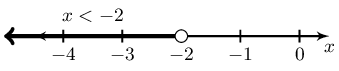In interval notation: $$(-\infty; -2)$$

$$3(x - 1) - 2 \le 6x + 4$$

\begin{align*} 3(x - 1) - 2 & \le 6x + 4 \\ 3x - 5 & \le 6x + 4 \\ 3x - 6x & \le 4 + 5 \\ -3x \le 9 \\ x \ge -\frac{9}{3} \\ x \ge -3 \end{align*}

Represented on a number line: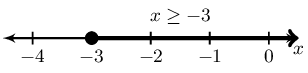In interval notation: $$[-3; \infty)$$

$$\dfrac{x - 7}{3} > \dfrac{2x - 3}{2}$$

\begin{align*} \frac{x - 7}{3} & > \frac{2x - 3}{2} \\ 2(x - 7) & > 3(2x - 3) \\ 2x - 14 > 6x - 9 \\ -4x > 5 \\ x < -\frac{5}{4} \end{align*}

Represented on a number line: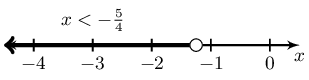In interval notation: $$(-\infty; -\frac{5}{4})$$

$$-4(x - 1) < x + 2$$

\begin{align*} -4(x - 1) & < x + 2 \\ -4x + 4 & < x + 2 \\ -5x < -2 \\ x > \frac{2}{5} \end{align*}

Represented on a number line: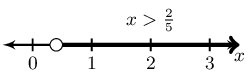In interval notation: $$(\frac{2}{5}; \infty)$$

$$\dfrac{1}{2}x + \dfrac{1}{3}(x - 1) \ge \dfrac{5}{6}x - \dfrac{1}{3}$$

\begin{align*} \frac{1}{2}x + \frac{1}{3}(x - 1) & \ge \frac{5}{6}x - \frac{1}{3} \\ \frac{1}{2}x + \frac{1}{3}x - \frac{1}{3} & \ge \frac{5}{6}x - \frac{1}{3} \\ \frac{1}{2}x + \frac{1}{3}x - \frac{5}{6}x & \ge \frac{1}{3} - \frac{1}{3} \\ \frac{3}{6}x + \frac{2}{6}x - \frac{5}{6}x & \ge 0 \\ 0x \ge 0 \end{align*}

The inequality is true for all real values of $$x$$.

$$-2 \le x - 1 < 3$$

$\begin{array}{ccccc} -2 & \le & x - 1 & < & 3 \\ -1 & \le & x & < & 4 \end{array}$

Represented on a number line: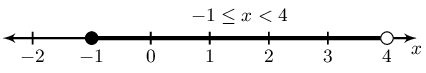In interval notation: $$[-1; 4)$$

$$-5 < 2x - 3 \le 7$$

$\begin{array}{ccccc} -5 & < & 2x - 3 & \le & 7 \\ -2 & < & 2x & \le & 10 \\ -1 & < & x & \le & 5 \end{array}$

Represented on a number line: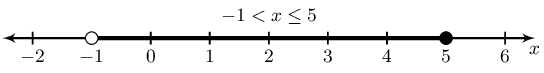In interval notation: $$(-1; 5]$$

$$7(3x + 2) - 5(2x - 3) > 7$$

\begin{align*} 7(3x + 2) - 5(2x - 3) & > 7 \\ 21x + 14 - 10x + 15 & > 7 \\ 11x & > -22 \\ x & > -2 \end{align*}

Represented on a number line: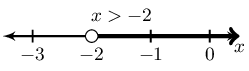In interval notation: $$(-2; \infty)$$

$$\dfrac{5x - 1}{-6} \ge \dfrac{1 - 2x}{3}$$

\begin{align*} \frac{5x - 1}{-6} & \ge \frac{1 - 2x}{3} \\ 5x - 1 & \ge -2(1 - 2x) \\ 5x - 1 & \ge -2 + 4x \\ 5x - 4x & \ge -1 \\ x & \ge -1 \end{align*}

Represented on a number line: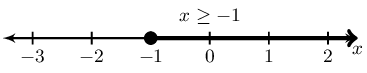In interval notation: $$[-1; \infty)$$

$$3 \le 4 - x \le 16$$

$\begin{array}{ccccc} 3 & \le & 4 - x & \le & 16 \\ -1 & \le & -x & \le & 12 \\ 1 & \ge & x & \ge & -12 \end{array}$

Represented on a number line: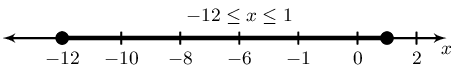In interval notation: $$[1; 12]$$

$$\dfrac{-7y}{3} - 5 > -7$$

\begin{align*} \frac{-7y}{3} - 5 & > -7 \\ -7y - 15 & > -21 \\ -7y & > -6 \\ y & < \frac{6}{7} \end{align*}

Represented on a number line: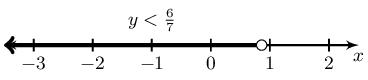In interval notation: $$(-\infty;\frac{6}{7})$$

$$1 \le 1 - 2y < 9$$

$\begin{array}{ccccc} 1 & \le & 1 - 2y & < & 9 \\ 0 & \le & -2y & < & 8 \\ 0 & \ge & y & > & -4 \\ -4 & < & y & \le & 0 \end{array}$

Represented on a number line: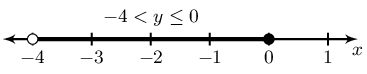In interval notation: $$(-4;0]$$

$$-2 < \dfrac{x - 1}{-3} < 7$$

$\begin{array}{ccccc} -2 & < & \dfrac{x - 1}{-3} & < & 7 \\ 6 & > & x - 1 & > & -21 \\ 7 & > & x & > & -20 \\ -20 & < & x & < & 7 \end{array}$

Represented on a number line: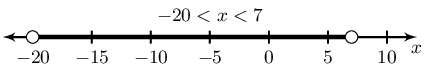In interval notation: $$(-20;7)$$

Solve for $$x$$ and show your answer in interval notation:

$$2x -1 < 3(x+11)$$

\begin{align*} 2 x -1 &< 3(x +11) \\ 2 x -1 &< 3 x +33 \\ 2 x -3 x &< 33 +1 \\ -1 x &< 34 \\ \therefore x &> -34 \end{align*}

$\left(-34;\infty\right)$

$$x -1 < -4(x-6)$$

\begin{align*} x -1 &< -4(x -6) \\ x -1 &< -4 x +24 \\ x +4 x &< 24 +1 \\ 5 x &< 25 \\ \therefore x &< 5 \end{align*}

$\left(-\infty;5\right)$

$$\dfrac{x-1}{8} \leq \dfrac{2(x-2)}{3}$$

\begin{align*} \frac{x-1}{8} &\leq \frac{2(x-2)}{3} \\ 3(x-1) &\leq 16(x-2) \\ 3x-3 &\leq 16x-32 \\ 3x -16x &\leq -32 +3 \\ -13x &\leq -29 \\ \therefore x &\geq\frac{29}{13} \end{align*}

$$\; x \in \left[ \frac{29}{13} ;\infty\right)$$.

$$\dfrac{x+2}{4} \leq \dfrac{-2(x-4)}{7}$$

\begin{align*} \frac{x+2}{4} &\leq \frac{-2(x-4)}{7} \\ 7(x+2) &\leq -8(x-4) \\ 7x+14 &\leq -8x+32 \\ 7x +8x &\leq 32 -14 \\ 15x &\leq 18 \\ \therefore x &\leq\frac{6}{5} \end{align*}

$$\; x \in \left(-\infty; \frac{6}{5} \right]$$.

$$\dfrac{1}{5}x - \dfrac{5}{4}(x+2) > \dfrac{1}{4}x + 3$$

\begin{align*} \frac{1}{5}x - \frac{5}{4}(x+2) &> \frac{1}{4}x +3 \\ 4x - 25(x+2) &> 5x +60 \\ 4x - 25 x-50 &> 5x +60 \\ 4x - 25 x -5x &> 60 + 50\\ -26x &> 110\\ \therefore x &< -\frac{55}{13} \end{align*}

The interval is: $\left(-\infty;-\frac{55}{13}\right)$

$$\dfrac{1}{5}x - \dfrac{2}{5}(x+3) \geq \dfrac{4}{2}x +3$$

\begin{align*} \frac{1}{5}x - \frac{2}{5}(x+3) &\geq \frac{4}{2}x +3 \\ 2x - 4(x+3) &\geq 20x +30 \\ 2x - 4 x-12 &\geq 20x +30 \\ 2x - 4 x -20x &\geq 30 + 12\\ -22x &\geq 42\\ \therefore x &\leq -\frac{21}{11} \end{align*}

The interval is: $\left(-\infty;-\frac{21}{11}\right]$

$$4x +3 < -3 \quad\text{or}\quad 4x +3 > 5$$

Solve the inequality: $\begin{array}{rclcrcl} 4x +3 &<& -3 &\text{or}& 4x +3 &>& 5 \\ 4x &<& -3-3 &\text{or}& 4x &>& 5-3 \\ x &<& \frac{-3-3}{4} &\text{or}& x &>& \frac{5-3}{4} \\ x &<& - \frac{3}{2} &\text{or}& x &>& \frac{1}{2} \\ \end{array}$

$\left(-\infty; - \frac{3}{2}\right) \cup \left(\frac{1}{2}; \infty\right)$

$$4 \ge -6x -6 \ge -3$$

Solve the inequality: $\begin{array}{rcccl} 4 &\ge& -6x -6 &\ge& -3 \\ 4+6 &\ge& -6x &\ge& -3+6 \\ \frac{4+6}{-6} &\le& x &\le& \frac{-3+6}{-6} \\ - \frac{5}{3} &\le& x &\le& - \frac{1}{2} \\ \end{array}$

$\left[- \frac{5}{3}; - \frac{1}{2}\right]$

Solve for the unknown variable and show your answer on a number line.

$$6b - 3 > b + 2 , ~b \in \mathbb{Z}$$
\begin{align*} 6b - 3 > b + 2 , ~b \in \mathbb{Z}\\ 5b > 5 \\ b > 1 \end{align*}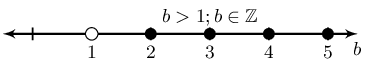$$3a - 1 < 4a + 6 , ~a \in \mathbb{N}$$
\begin{align*} 3a - 1 < 4a + 6 \\ -a < 7 \\ a > -7 \end{align*}

However we are told that $$a \in \mathbb{N}$$ and so $$a > 0$$.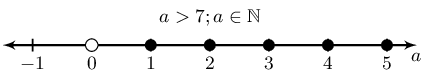$$\dfrac{b-3}{2} + 1 < \dfrac{b}{4} - 4 , ~b \in \mathbb{R}$$
\begin{align*} \frac{b-3}{2} + 1 < \frac{b}{4} - 4 \\ 2b - 6 + 4 < b - 16 \\ b < -14 \end{align*}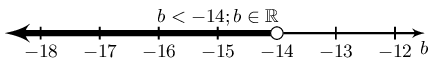$$\dfrac{4a +7}{3} - 5 > a - \dfrac{2}{3} , ~a \in \mathbb{N}$$
\begin{align*} \frac{4a +7}{3} - 5 > a - \frac{2}{3} \\ 4a + 7 - 15 > 3a - 2 \\ a > 6 \end{align*}